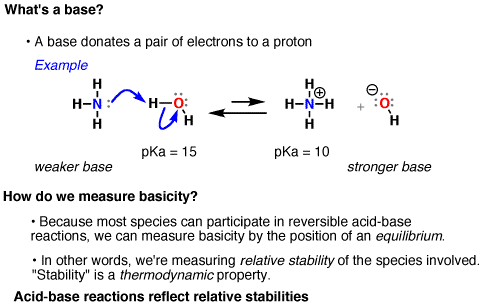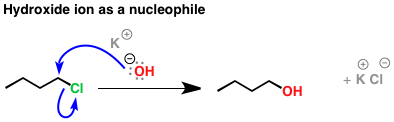# 6.10: Lewis Bases as Nucleophilic Reactants in Organic Reactions

$$\newcommand{\vecs}{\overset { \rightharpoonup} {\mathbf{#1}} }$$ $$\newcommand{\vecd}{\overset{-\!-\!\rightharpoonup}{\vphantom{a}\smash {#1}}}$$$$\newcommand{\id}{\mathrm{id}}$$ $$\newcommand{\Span}{\mathrm{span}}$$ $$\newcommand{\kernel}{\mathrm{null}\,}$$ $$\newcommand{\range}{\mathrm{range}\,}$$ $$\newcommand{\RealPart}{\mathrm{Re}}$$ $$\newcommand{\ImaginaryPart}{\mathrm{Im}}$$ $$\newcommand{\Argument}{\mathrm{Arg}}$$ $$\newcommand{\norm}{\| #1 \|}$$ $$\newcommand{\inner}{\langle #1, #2 \rangle}$$ $$\newcommand{\Span}{\mathrm{span}}$$ $$\newcommand{\id}{\mathrm{id}}$$ $$\newcommand{\Span}{\mathrm{span}}$$ $$\newcommand{\kernel}{\mathrm{null}\,}$$ $$\newcommand{\range}{\mathrm{range}\,}$$ $$\newcommand{\RealPart}{\mathrm{Re}}$$ $$\newcommand{\ImaginaryPart}{\mathrm{Im}}$$ $$\newcommand{\Argument}{\mathrm{Arg}}$$ $$\newcommand{\norm}{\| #1 \|}$$ $$\newcommand{\inner}{\langle #1, #2 \rangle}$$ $$\newcommand{\Span}{\mathrm{span}}$$

The basis of chemistry is that opposite charges attract and like charges repel, and that in reactions, electrons flow from “electron rich” areas to “electron poor” areas. A name to the types of species that are considered electron rich and “electron poor”. They are called nucleophiles and electrophiles.

## Nucleophiles

A nucleophiles (from “nucleus loving”, or “positive-charge loving”) is a reactant that provides a pair of electrons to form a new covalent bond. This is the exact definition of a Lewis base discussed earlier. Hence, nucleophiles are Lewis bases. When the nucleophile donates a pair of electrons to a proton, it’s called a Brønsted base, or simply, “base”. As you can see, nucleophiles all have pairs of electrons to donate, and tend to be rich in electrons. Here are some examples of Lewis bases you are probably familiar with.Figure $$\PageIndex{1}$$: Examples of Lewis bases

When the nucleophile donates a pair of electrons to a proton, it’s called a Brønsted base.

How is nucleophilicity different from basicity? Well, since it’s not limited to simply forming a bond to hydrogen anymore, this leads to some extra complications. Let’s just talk about the measurement problem first. Many reactions of nucleophiles are not reversible. A bond forms, a bond breaks, and that’s the end of the reaction. The problem with this from a measurement standpoint is that we often cannot determine an equilibrium constant for a reaction. And if we can’t do that, then we cannot develop a reactivity scale based on equilibria. If we can’t measure equilibria, then what do we do? Well, we use the next best measurement available: to measure reaction rates, but that is discuss in the following chapters.Figure $$\PageIndex{2}$$: A nucleophilic reaction with a nucleophile donating a pair of electrons to the the carbon

Example $$\PageIndex{1}$$: Basicity in Organic Chemistry Reactions

So how do reactions of nucleophiles at hydrogen differ from reactions of nucleophiles at carbon? Well, they’re more easily reversible, for one thing. We can measure acidity (and, by extension, basicity) through the measure known as pKa, which is a reflection of the position of the equilibrium between an acid and its conjugate base.We can vaguely define “nucleophilicity” as “the extent to which a species can donate a pair of electrons”. A more precise definition will be discussed in organic chemistry courses.

## Electrophilicity

Similarly, the extent to which a species can accept a lone pair of electrons is called “electrophilicity”. An electrophile (from “electron-loving”, or “negative-charge loving”) is a species that accepts a pair of electrons to form a new covalent bond. Again, this should sound familiar: this is the definition of a Lewis acid. An electrophile that accepts an electron pair at hydrogen is called a Brønsted acid, or just “acid”. Here are some examples of Lewis acids you’re familiar with.Figure $$\PageIndex{3}$$﻿﻿: Lewis acids

Lewis acidity is essentially electrophilicity. Acidity (Brønsted acidity) is what we call it when the electrophile is a proton.

Example $$\PageIndex{1}$$: The Hydroxide Ion

When hydroxide ion donates a pair of electrons to an electrophilic atom (such as carbon here) to form a new covalent bond, it is acting as a nucleophile.﻿

And as we’ve seen before, when hydroxide ion donates a pair of electrons to an (acidic) proton to form a new covalent bond, we say it’s acting as a “base”.

﻿﻿

The vast majority of the reactions you’ll see in organic chemistry (>95%) – will be reactions where a nucleophile donates a pair of electrons to an electrophile (i.e., a nucleophile attacks an electrophile) with very few exceptions! This is why understanding where electrons are, and how electrons flow is so important – because the electron richness (or poorness) of an atom (or molecule) determines its nucleophilicity or electrophilicity, which in turn determines its reactivity. It’s not an exaggeration to say that nucleophilicity and electrophilicity (and hence Lewis Acids and Bases) are the fundamental basis of chemical reactivity.

## Summary

Basicity is a subset of nucleophilicity. While, all nucleophiles are Lewis bases since they donate a lone pair of electrons. A (Brønsted) base is the name we give to a nucleophile since it forms a bond to a proton ($$H^+$$). To summarize, when we’re talking about basicity and nucleophilicity, we’re talking about these two types of events.